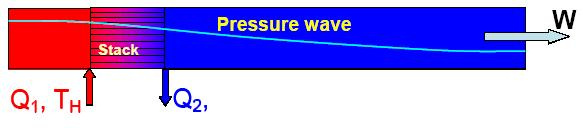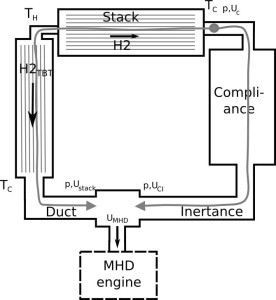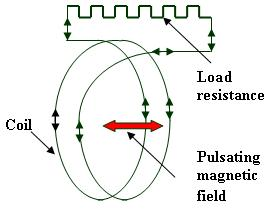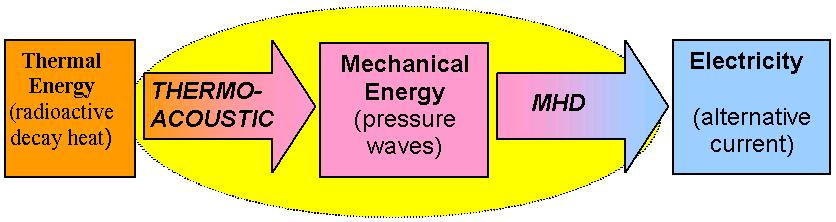# Method

THERMOACOUSTIC

The thermo-acoustic effect is a thermodynamic process allowing transforming heat into mechanical energy. The engine based on this principle is extremely simple, even if the mathematical model is not so easy, and the physic difficult to explainFigure 1: Schematic representation of a standing wave thermo acoustic engine

The main element of the engine is a heat exchanger with an extremely high heat exchange surface: the stack. This exchanger can be placed in either a linear tube or a loop. When a temperature difference greater than a critical value is imposed on the both ends of the stack, a pressure wave is spontaneously generated which propagates in the tube at the speed of sound. The amplitude of this pressure wave depends of many factors such as: geometry of the engine and physical properties of the fluid and the stack, temperature gradient along the stack and mean pressure of the thermodynamic fluid. It is admitted that, in good conditions, the amplitude of the pressure oscillation can reach up to 10% of the imposed mean pressure. The best fluid generally invoked in the literature seems to be helium due to its relatively high value of the heat transfer coefficient. Based on this principle two types of engine can be considered:

1- A standing wave engine using a linear tube as it can be seen in the figure 1. In this case the wave is oscillating in a tube and the mechanical power which is proportional to the scalar product of the oscillating pressure by the oscillating velocity vanishes if the tube is closed by rigid wall, but takes some value if a proper system of energy transfer is applied;

2- The travelling wave concept (Figure 2) uses a close loop in which the thermo-acoustic wave propagates continuously. In this case, a new element, the thermo-buffer tube must be added to adjust the temperature at the hot and cold sources. This case seems to be better on the thermodynamics point of view because it is more reversible than the standing wave concept.Figure 2: Schematic representation of a travelling wave thermo acoustic engine

MHD GENERATOR

The problem now is to convert the mechanical energy supplied in the form of a vibration into electrical energy. An MHD generator could be used to realize this function. The process uses the induction mechanism which is represented schematically by the figure 3.Figure 3: Principle of the inductive MHD generator

When a pulsating magnetic field is applied through a closed coil, the magnetic flux variation induces an electric current in the electrical circuit of the coil able to supply the load represented here by an electrical resistance.

To produce the pulsating magnetic field, the process uses the interaction of an imposed DC magnetic field with the pulsating liquid metal flow generated by the thermo-acoustic effect. A permanent magnet produces a DC magnetic field which interacts with a pulsating electro-conducting media generating an induced AC electrical current circulating in the electro-conducting media. This AC current generates itself an induced AC magnetic field which produces a magnetic flux variation producing the induced current which is able to supply a load.
*The main parameters that were adopted for the calculation and further for the design of the TA/MHD generators are the following:

Temperature of the hot source Temperature of the cold source Melting point of the sodium Carnot efficiency Global expected efficiency Level of electrical power Heat source power
1100 K400 K 373 K0.64~ 0,25 200 W~1000 W